Documentation

## Visualize RF Impairments

Apply various RF impairments to a QAM signal. Observe the effects by using constellation diagrams, time-varying error vector magnitude (EVM) plots, and spectrum plots. Estimate the equivalent signal-to-noise ratio (SNR).

### Initialization

Set the sample rate, modulation order, and SNR. Calculate the reference constellation points.

```fs = 1000; M = 16; snrdB = 30; refConst = qammod(0:M-1,M,'UnitAveragePower',true); ```

Create constellation diagram and time scope objects to visualize the impairment effects.

```constDiagram = comm.ConstellationDiagram('ReferenceConstellation',refConst); timeScope = dsp.TimeScope('YLimits',[0 40],'SampleRate',fs,'TimeSpan',1, ... 'ShowGrid',true,'YLabel','EVM (%)'); ```

### White Noise

Generate a 16-QAM signal, and pass it through an AWGN channel. Plot its constellation.

```data = randi([0 M-1],1000,1); modSig = qammod(data,M,'UnitAveragePower',true); noisySig = awgn(modSig,snrdB); constDiagram(noisySig) ```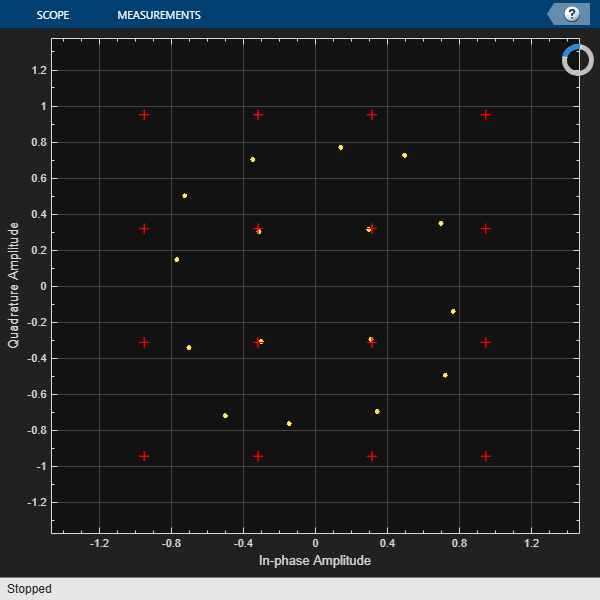Estimate the EVM of the noisy signal from the reference constellation points.

```evm = comm.EVM('ReferenceSignalSource','Estimated from reference constellation', ... 'ReferenceConstellation',refConst, ... 'Normalization','Average constellation power'); rmsEVM = evm(noisySig) ```
```rmsEVM = 3.1768 ```

The modulation error rate (MER) closely corresponds to the SNR. Create an MER object, and estimate the SNR.

```mer = comm.MER('ReferenceSignalSource','Estimated from reference constellation', ... 'ReferenceConstellation',refConst); snrEst = mer(noisySig) ```
```snrEst = 30.1071 ```

The estimate is quite close to the specified SNR of 30 dB.

### Amplifier Distortion

Create an amplifier using the memoryless nonlinearity object.

```amp = comm.MemorylessNonlinearity('IIP3',38,'AMPMConversion',0); ```

Pass the modulated signal through the nonlinear amplifier, and plot its constellation diagram.

```txSig = amp(modSig); constDiagram(txSig) ```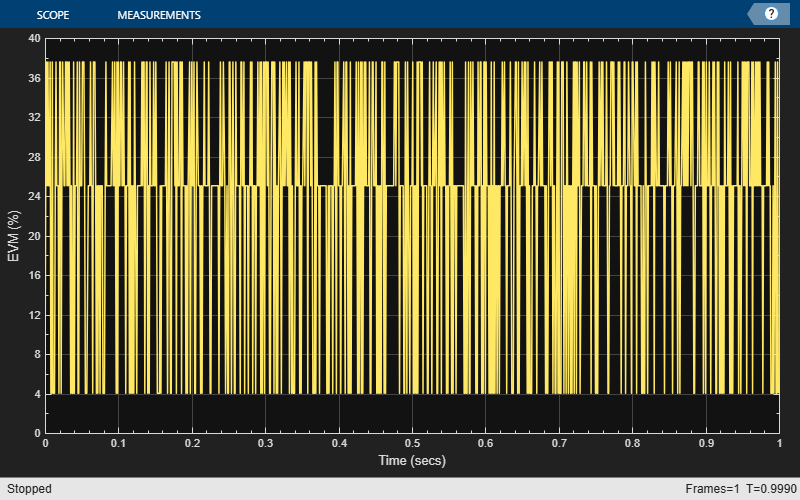The corner points of the constellation have moved toward the origin due to amplifier gain compression.

Introduce a small AM/PM conversion, and display the received signal constellation.

```amp.AMPMConversion = 1; txSig = amp(modSig); constDiagram(txSig) ```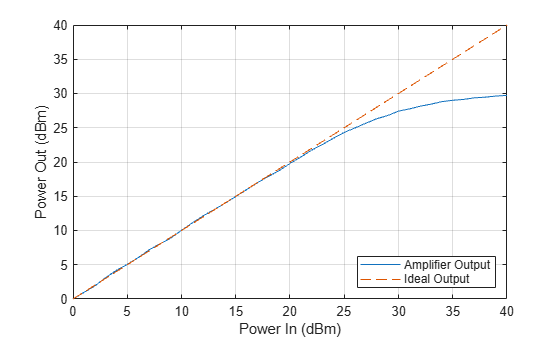The constellation has rotated due to the AM/PM conversion. To compute the time-varying EVM, release the EVM object and set the `AveragingDimensions` property to 2. To estimate the EVM against an input signal, omit the `ReferenceSignalSource` property definition. This method produces more accurate results.

```evm = comm.EVM('AveragingDimensions',2); evmTime = evm(modSig,txSig); ```

Plot the time-varying EVM of the distorted signal.

```timeScope(evmTime) ```Compute the RMS EVM.

```evmRMS = sqrt(mean(evmTime.^2)) ```
```evmRMS = 35.5919 ```

Compute the MER.

```mer = comm.MER; snrEst = mer(modSig,txSig) ```
```snrEst = 8.1392 ```

The SNR (≈8 dB) is reduced from its initial value (∞) due to amplifier distortion.

Specify input power levels ranging from 0 to 40 dBm. Convert those levels to their linear equivalent in W. Initialize the output power vector.

```powerIn = 0:40; pin = 10.^((powerIn-30)/10); powerOut = zeros(length(powerIn),1); ```

Measure the amplifier output power for the range of input power levels.

```for k = 1:length(powerIn) data = randi([0 15],1000,1); txSig = qammod(data,16,'UnitAveragePower',true)*sqrt(pin(k)); ampSig = amp(txSig); powerOut(k) = 10*log10(var(ampSig))+30; end ```

Plot the power output versus power input curve.

```figure plot(powerIn,powerOut,powerIn,powerIn,'--') legend('Amplifier Output','Ideal Output','location','se') xlabel('Power In (dBm)') ylabel('Power Out (dBm)') grid ```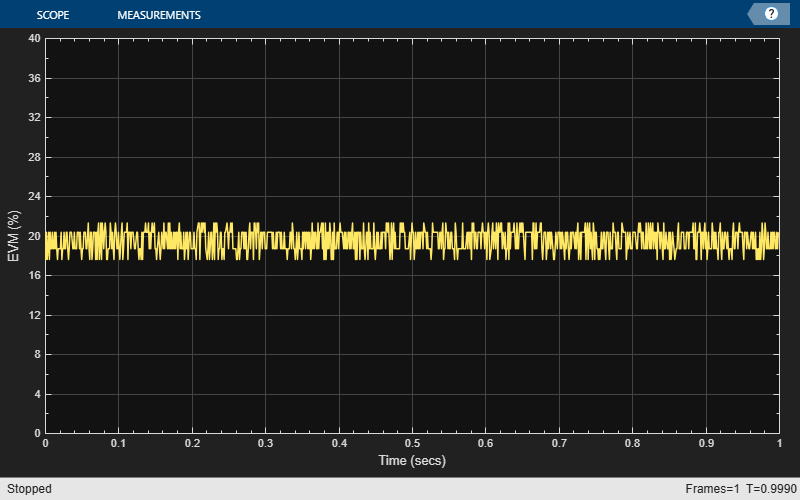The output power levels off at 30 dBm. The amplifier exhibits nonlinear behavior for input power levels greater than 25 dBm.

### I/Q Imbalance

Apply an amplitude and phase imbalance to the modulated signal.

```ampImb = 3; phImb = 10; gainI = 10.^(0.5*ampImb/20); gainQ = 10.^(-0.5*ampImb/20); imbI = real(modSig)*gainI*exp(-0.5i*phImb*pi/180); imbQ = imag(modSig)*gainQ*exp(1i*(pi/2 + 0.5*phImb*pi/180)); rxSig = imbI + imbQ; ```

```constDiagram(rxSig) ```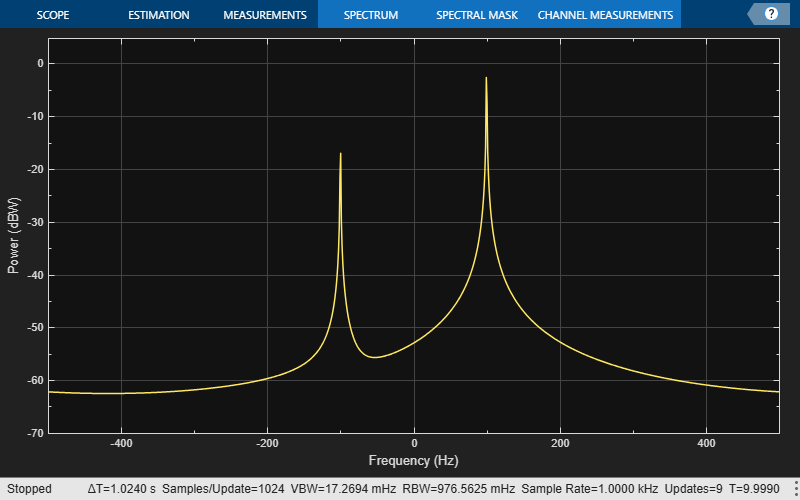The magnitude and phase of the constellation has changed as a result of the I/Q imbalance.

Calculate and plot the time-varying EVM.

```evmTime = evm(modSig,rxSig); timeScope(evmTime) ```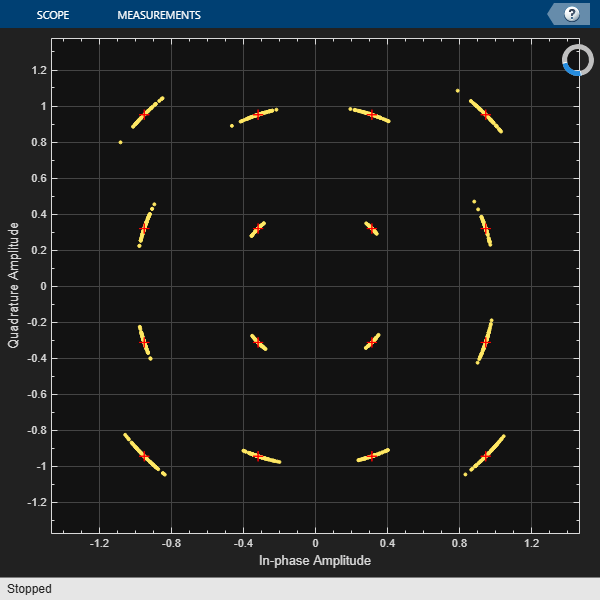The EVM exhibits a behavior that is similar to that experienced with a nonlinear amplifier though the variance is smaller.

Create a 100 Hz sine wave having a 1000 Hz sample rate.

```sinewave = dsp.SineWave('Frequency',100,'SampleRate',1000, ... 'SamplesPerFrame',1e4,'ComplexOutput',true); x = sinewave(); ```

Apply the same 3 dB and 10 degree I/Q imbalance.

```ampImb = 3; phImb = 10; gainI = 10.^(0.5*ampImb/20); gainQ = 10.^(-0.5*ampImb/20); imbI = real(x)*gainI*exp(-0.5i*phImb*pi/180); imbQ = imag(x)*gainQ*exp(1i*(pi/2 + 0.5*phImb*pi/180)); y = imbI + imbQ; ```

Plot the spectrum of the imbalanced signal.

```spectrum = dsp.SpectrumAnalyzer('SampleRate',1000,'PowerUnits','dBW'); spectrum(y) ```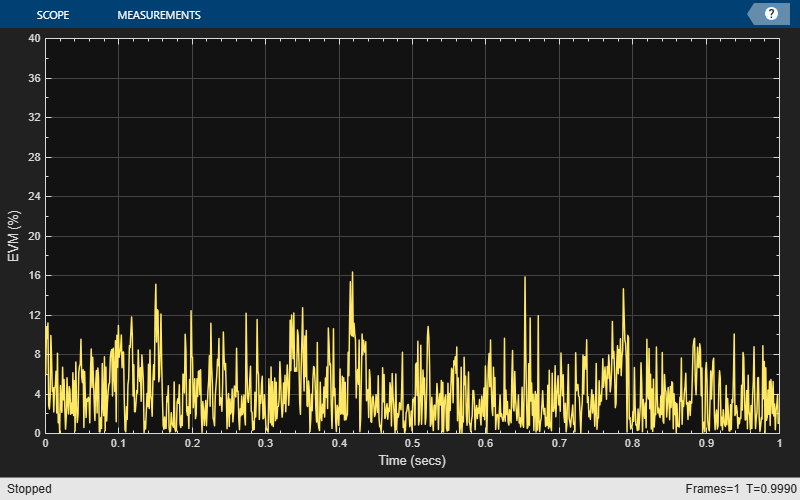The I/Q imbalance introduces a second tone at -100 Hz, which is the inverse of the input tone.

### Phase Noise

Apply phase noise to the transmitted signal. Plot the resulting constellation diagram.

```pnoise = comm.PhaseNoise('Level',-50,'FrequencyOffset',20,'SampleRate',fs); pnoiseSig = pnoise(modSig); constDiagram(pnoiseSig) ```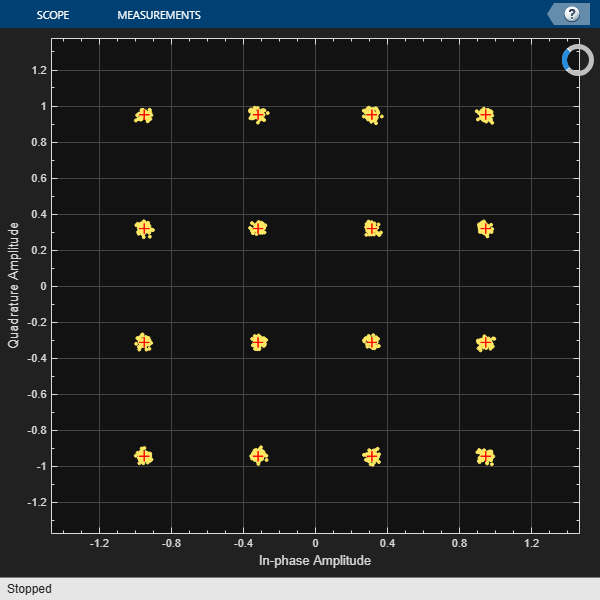The phase noise introduces a rotational jitter.

Compute and plot the EVM of the received signal.

```evmTime = evm(modSig,pnoiseSig); timeScope(evmTime) ```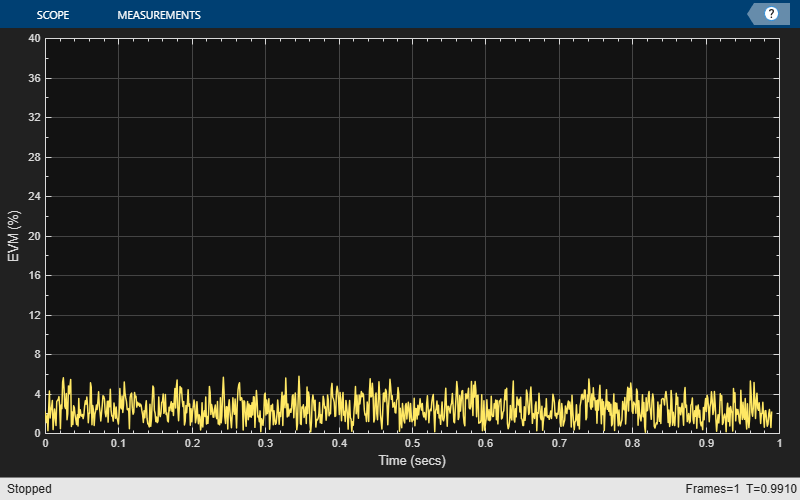Determine the RMS EVM.

```evmRMS = sqrt(mean(evmTime.^2)) ```
```evmRMS = 6.1989 ```

### Filter Effects

Specify the samples per symbol parameter. Create a pair of raised cosine matched filters.

```sps = 4; txfilter = comm.RaisedCosineTransmitFilter('RolloffFactor',0.2,'FilterSpanInSymbols',8, ... 'OutputSamplesPerSymbol',sps,'Gain',sqrt(sps)); rxfilter = comm.RaisedCosineReceiveFilter('RolloffFactor',0.2,'FilterSpanInSymbols',8, ... 'InputSamplesPerSymbol',sps,'Gain',1/sqrt(sps), ... 'DecimationFactor',sps); ```

Determine the delay through the matched filters.

```fltDelay = 0.5*(txfilter.FilterSpanInSymbols + rxfilter.FilterSpanInSymbols); ```

Pass the modulated signal through the matched filters.

```filtSig = txfilter(modSig); rxSig = rxfilter(filtSig); ```

To account for the delay through the filters, discard the first `fltDelay` samples.

```rxSig = rxSig(fltDelay+1:end); ```

To accommodate the change in the number of received signal samples, create new constellation diagram and time scope objects.

```constDiagram = comm.ConstellationDiagram('ReferenceConstellation',refConst); timeScope = dsp.TimeScope('YLimits',[0 40],'SampleRate',fs,'TimeSpan',1, ... 'ShowGrid',true,'YLabel','EVM (%)'); ```

Estimate EVM. Plot the received signal constellation diagram and the time-varying EVM.

```evm = comm.EVM('ReferenceSignalSource','Estimated from reference constellation', ... 'ReferenceConstellation',refConst, ... 'Normalization','Average constellation power','AveragingDimensions',2); evmTime = evm(rxSig); constDiagram(rxSig) timeScope(evmTime) ```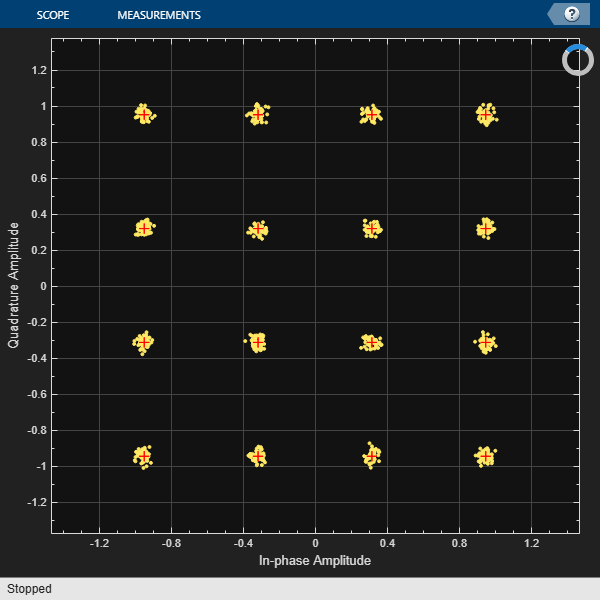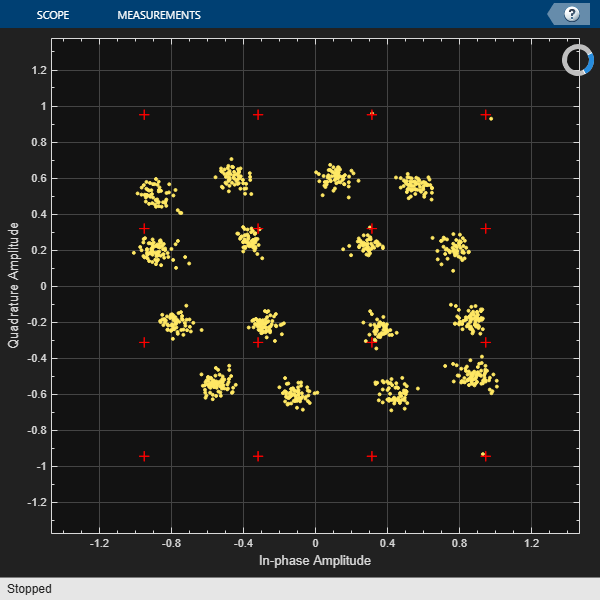Determine the RMS EVM.

```evmRMS = sqrt(mean(evmTime.^2)) ```
```evmRMS = 2.7199 ```

Determine the equivalent SNR.

```mer = comm.MER; snrEst = mer(modSig(1:end-fltDelay),rxSig) ```
```snrEst = 31.4603 ```

### Combined Effects

Combine the effects of the filters, nonlinear amplifier, AWGN, and phase noise. Display the constellation and EVM diagrams.

Create EVM, time scope and constellation diagram objects.

```evm = comm.EVM('ReferenceSignalSource','Estimated from reference constellation', ... 'ReferenceConstellation',refConst, ... 'Normalization','Average constellation power','AveragingDimensions',2); timeScope = dsp.TimeScope('YLimits',[0 40],'SampleRate',fs,'TimeSpan',1, ... 'ShowGrid',true,'YLabel','EVM (%)'); constDiagram = comm.ConstellationDiagram('ReferenceConstellation',refConst); ```

Specify the nonlinear amplifier and phase noise objects.

```amp = comm.MemorylessNonlinearity('IIP3',45,'AMPMConversion',0); pnoise = comm.PhaseNoise('Level',-55,'FrequencyOffset',20,'SampleRate',fs); ```

Filter and then amplify the modulated signal.

```txfiltOut = txfilter(modSig); txSig = amp(txfiltOut); ```

Add phase noise. Pass the impaired signal through the AWGN channel. Plot the constellation diagram.

```rxSig = awgn(txSig,snrdB); pnoiseSig = pnoise(rxSig); rxfiltOut = rxfilter(pnoiseSig); constDiagram(rxfiltOut) ```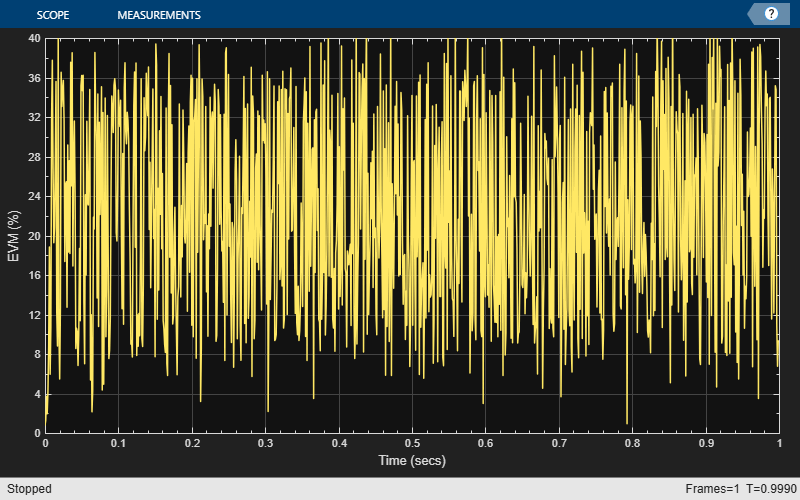Calculate the time-varying EVM. Plot the result.

```evmTime = evm(rxfiltOut); timeScope(evmTime) ```Determine the RMS EVM.

```evmRMS = sqrt(mean(evmTime.^2)) ```
```evmRMS = 6.6444 ```

Estimate the SNR.

```mer = comm.MER('ReferenceSignalSource','Estimated from reference constellation', ... 'ReferenceConstellation',refConst); snrEst = mer(rxfiltOut) ```
```snrEst = 23.6978 ```

This value is approximately 6 dB worse than the specified value of 30 dB, which means that the effects of the other impairments are significant and will degrade the bit error rate performance.